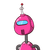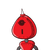# the sum of two integers is -278. if one of the integers is -156, find the other

the sum of two integers is -278. if one of the integers is -156, find the other

### 2 thoughts on “the sum of two integers is -278. if one of the integers is -156, find the other”

1.-156+x=-278

X=-278+156

X= – 122 ( – 278 is greater than 156 so negative sign)

2.-122

Step-by-step explanation:

Sum of integers: 278

One integer: 156

Other integer: x

According to question:

x+(156)= 278

x = 278 + 156

= 122